# CSC 3130 Formal Languages and Automata Theory Tutorial

• Slides: 20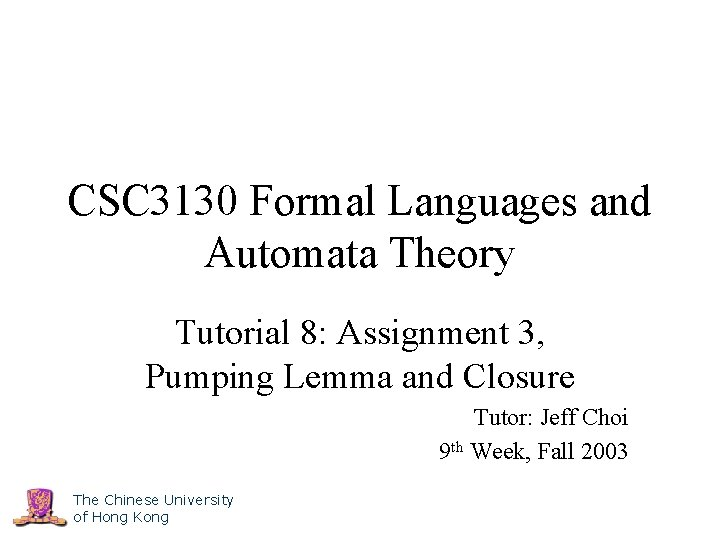CSC 3130 Formal Languages and Automata Theory Tutorial 8: Assignment 3, Pumping Lemma and Closure Tutor: Jeff Choi 9 th Week, Fall 2003 The Chinese University of Hong KongQuestion 1 • Construction of CFG • Hints: – Think about the inductive definition, see Tutorial 6 for examples – Recognize the substrings that each variable represent – Be careful of “extreme cases” e. g. Does ε belongs to the language? What if an and n = 0? – Be careful of the “special case” pitfall e. g. the set of all strings over {0, 1} with equal number of 0’s and 1’s, 0 n 1 n is only a special case The Chinese University of Hong Kong 2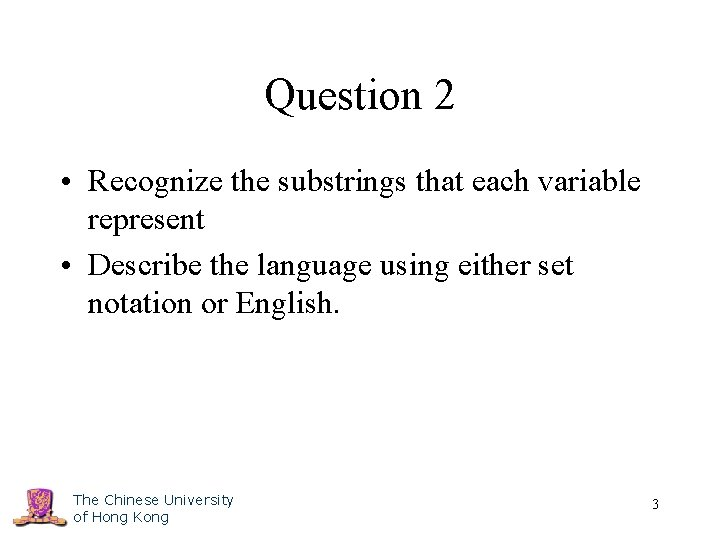Question 2 • Recognize the substrings that each variable represent • Describe the language using either set notation or English. The Chinese University of Hong Kong 3Question 3 • Show a string that has multiple leftmost derivations from the start symbol • Equivalently: multiple rightmost derivations or multiple parse trees • Making the CFG unambiguous, see Tutorial 6 for example • Explain briefly why you have a unique parse tree for any strings accepted by your unambiguous CFG The Chinese University of Hong Kong 4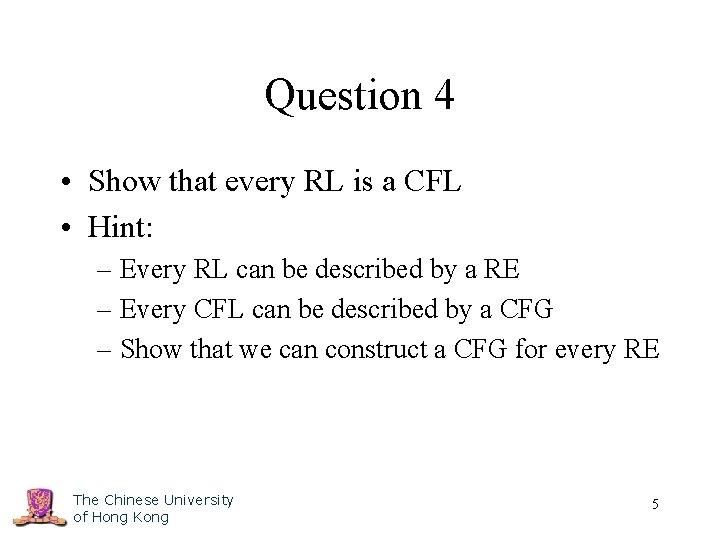Question 4 • Show that every RL is a CFL • Hint: – Every RL can be described by a RE – Every CFL can be described by a CFG – Show that we can construct a CFG for every RE The Chinese University of Hong Kong 5Question 5 • See Tutorial 7 for example on conversion into CNF and GNF • Make sure there is no -production nor unit-production The Chinese University of Hong Kong 6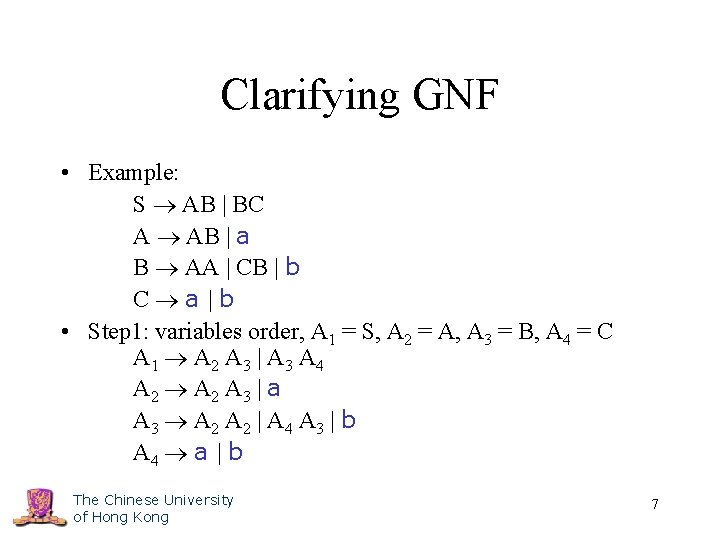Clarifying GNF • Example: S AB | BC A AB | a B AA | CB | b C a |b • Step 1: variables order, A 1 = S, A 2 = A, A 3 = B, A 4 = C A 1 A 2 A 3 | A 3 A 4 A 2 A 3 | a A 3 A 2 | A 4 A 3 | b A 4 a | b The Chinese University of Hong Kong 7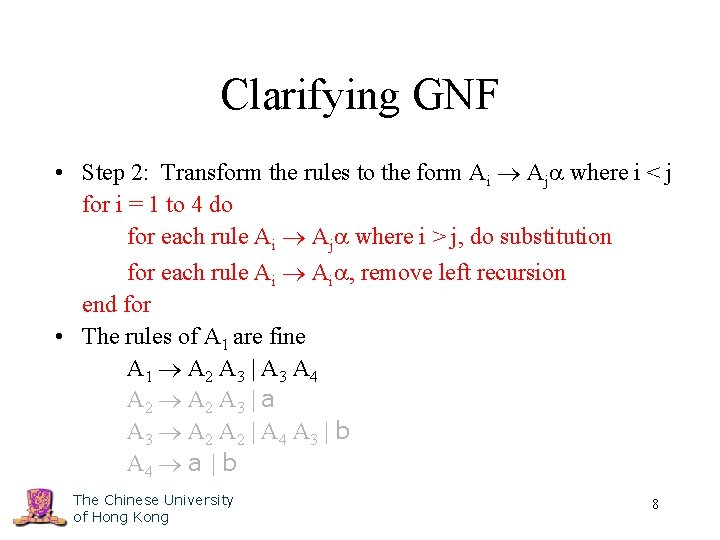Clarifying GNF • Step 2: Transform the rules to the form Ai Aj where i < j for i = 1 to 4 do for each rule Ai Aj where i > j, do substitution for each rule Ai , remove left recursion end for • The rules of A 1 are fine A 1 A 2 A 3 | A 3 A 4 A 2 A 3 | a A 3 A 2 | A 4 A 3 | b A 4 a | b The Chinese University of Hong Kong 8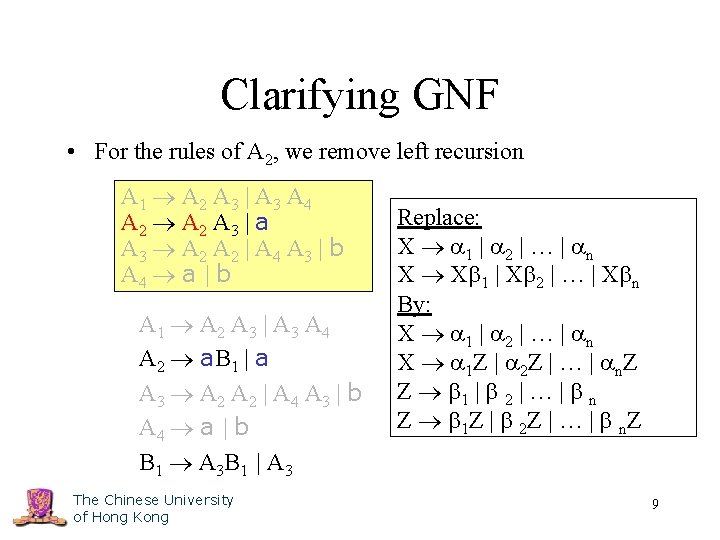Clarifying GNF • For the rules of A 2, we remove left recursion A 1 A 2 A 3 | A 3 A 4 A 2 A 3 | a A 3 A 2 | A 4 A 3 | b A 4 a | b A 1 A 2 A 3 | A 3 A 4 A 2 a. B 1 | a A 3 A 2 | A 4 A 3 | b A 4 a | b B 1 A 3 B 1 | A 3 The Chinese University of Hong Kong Replace: X 1 | 2 | … | n X X 1 | X 2 | … | X n By: X 1 | 2 | … | n X 1 Z | 2 Z | … | n. Z Z 1 | 2 | … | n Z 1 Z | 2 Z | … | n. Z 9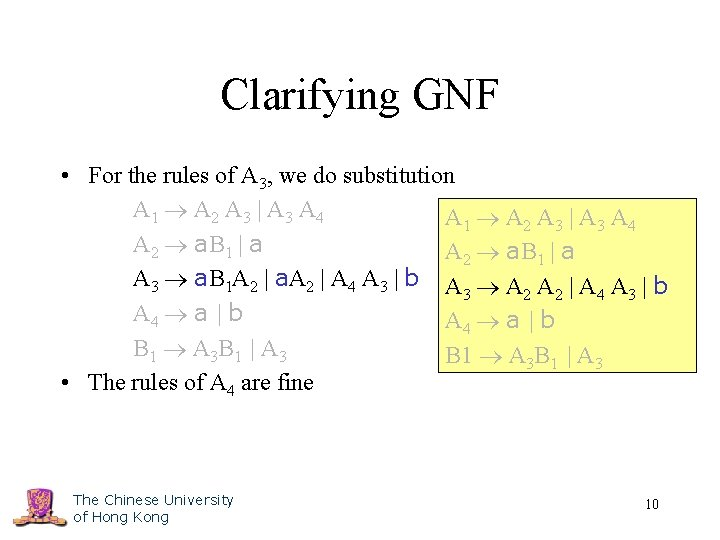Clarifying GNF • For the rules of A 3, we do substitution A 1 A 2 A 3 | A 3 A 4 A 2 a. B 1 | a A 3 a. B 1 A 2 | a. A 2 | A 4 A 3 | b A A A | b 3 2 2 4 3 A 4 a | b B 1 A 3 B 1 | A 3 • The rules of A 4 are fine The Chinese University of Hong Kong 10Clarifying GNF • Step 3: substitute backward, A 4, A 3, A 2, A 1, B 1 A 1 a. B 1 A 3 | a. A 3 A 1 A 2 A 3 | A 3 A 4 | a. B 1 A 2 A 4 | a. A 2 A 4 A 2 a. B 1 | a. A 3 A 4 | b. A 4 A 3 a. B 1 A 2 | a. A 2 | A 4 A 3 | b A 2 a. B 1 | a A 4 a | b A 3 a. B 1 A 2 | a. A 3 | b B 1 A 3 B 1 | A 3 A 4 a | b B 1 a. B 1 A 2 B 1 | a. A 3 B 1 | b. B 1 | a. B 1 A 2 | a. A 3 | b The Chinese University of Hong Kong 11Simpler Variable Ordering • Example: S AB | BC A AB | a B AA | CB | b C a |b • Simpler variables order, A 1 = S, A 2 = B, A 3 = A, A 4 = C A 1 A 3 A 2 | A 2 A 4 A 2 A 3 A 3 | A 4 A 2 | b A 3 A 2 | a A 4 a | b The Chinese University of Hong Kong 12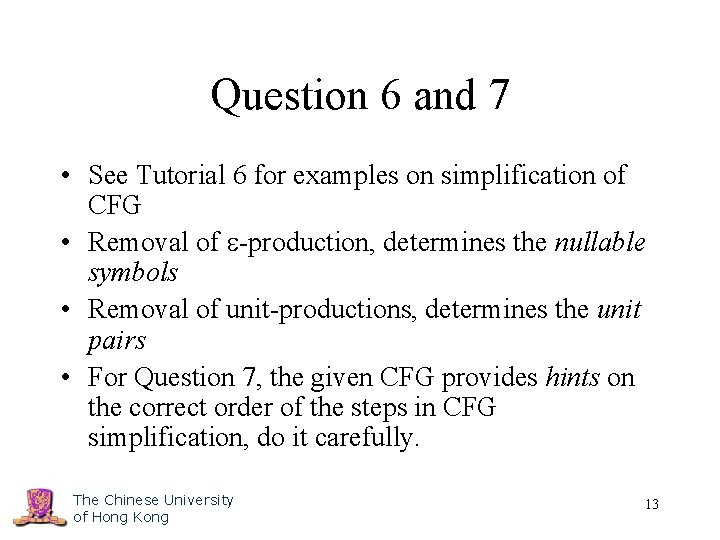Question 6 and 7 • See Tutorial 6 for examples on simplification of CFG • Removal of -production, determines the nullable symbols • Removal of unit-productions, determines the unit pairs • For Question 7, the given CFG provides hints on the correct order of the steps in CFG simplification, do it carefully. The Chinese University of Hong Kong 13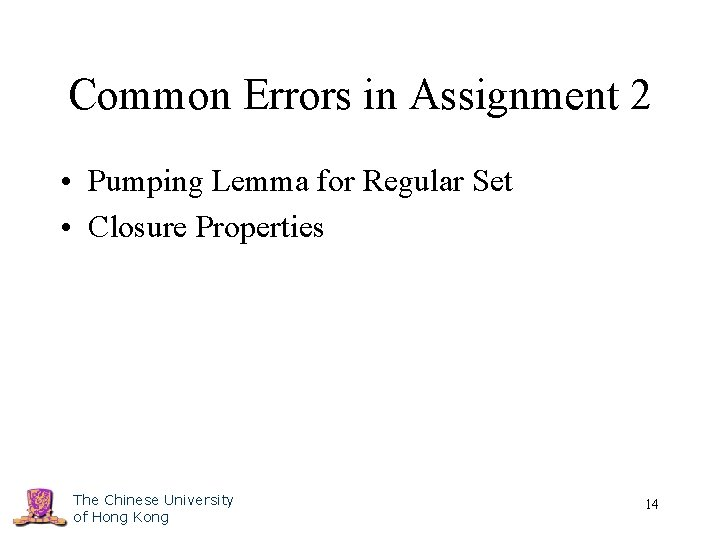Common Errors in Assignment 2 • Pumping Lemma for Regular Set • Closure Properties The Chinese University of Hong Kong 14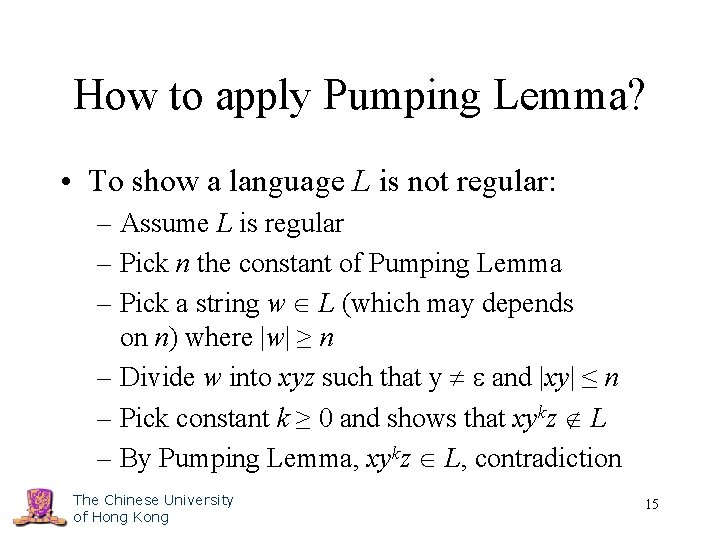How to apply Pumping Lemma? • To show a language L is not regular: – Assume L is regular – Pick n the constant of Pumping Lemma – Pick a string w L (which may depends on n) where |w| ≥ n – Divide w into xyz such that y and |xy| ≤ n – Pick constant k ≥ 0 and shows that xykz L – By Pumping Lemma, xykz L, contradiction The Chinese University of Hong Kong 15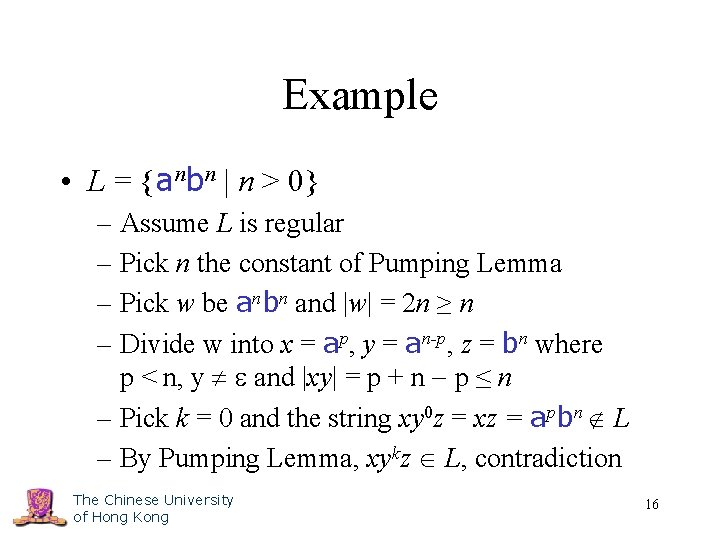Example • L = {anbn | n > 0} – Assume L is regular – Pick n the constant of Pumping Lemma – Pick w be anbn and |w| = 2 n ≥ n – Divide w into x = ap, y = an-p, z = bn where p < n, y and |xy| = p + n p ≤ n – Pick k = 0 and the string xy 0 z = xz = apbn L – By Pumping Lemma, xykz L, contradiction The Chinese University of Hong Kong 16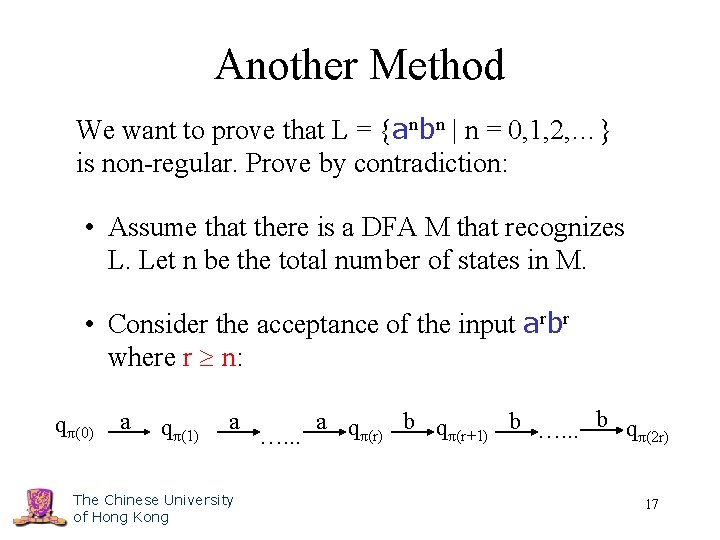Another Method We want to prove that L = {anbn | n = 0, 1, 2, …} is non-regular. Prove by contradiction: • Assume that there is a DFA M that recognizes L. Let n be the total number of states in M. • Consider the acceptance of the input arbr where r n: qπ(0) a qπ(1) a The Chinese University of Hong Kong a qπ(r) b qπ(r+1) b …. . . b q π(2 r) …. . . 17Another Method • Since r n and M has only n states, there must be at least one state visited twice in the first r transitions. Let this state be visited at the ith and the jth steps, where j > i and (j – i) ≥ 1. qπ(i) = qπ(j) qπ(0) qπ(2 r) F The path in M when reading arbr • By skipping the loop, ar-(j-i)br should also be accepted by M, but this is contradictory since ar-(j-i)br L The Chinese University of Hong Kong 18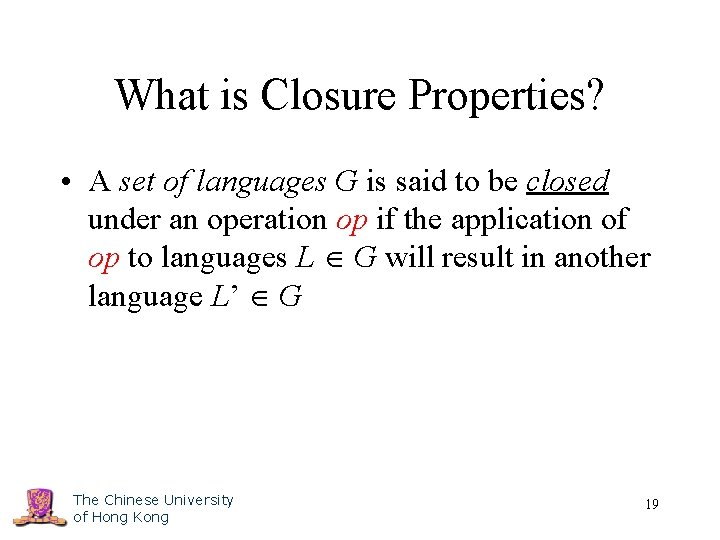What is Closure Properties? • A set of languages G is said to be closed under an operation op if the application of op to languages L G will result in another language L’ G The Chinese University of Hong Kong 19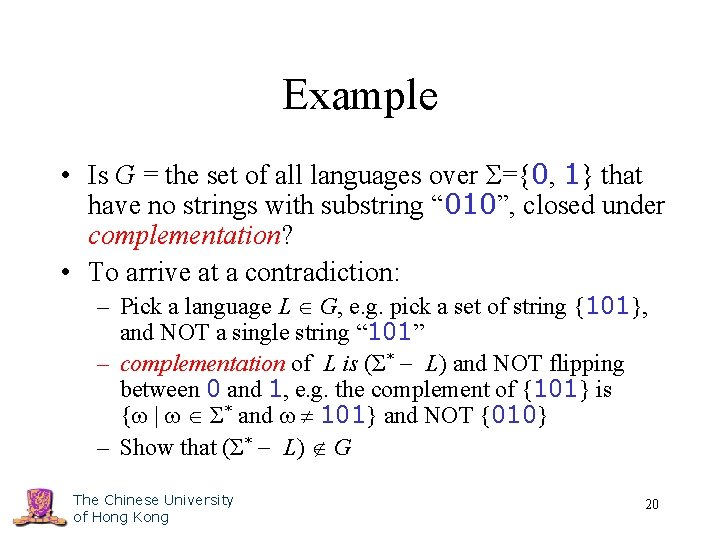Example • Is G = the set of all languages over Σ={0, 1} that have no strings with substring “ 010”, closed under complementation? • To arrive at a contradiction: – Pick a language L G, e. g. pick a set of string {101}, and NOT a single string “ 101” – complementation of L is (Σ* L) and NOT flipping between 0 and 1, e. g. the complement of {101} is { | Σ* and 101} and NOT {010} – Show that (Σ* L) G The Chinese University of Hong Kong 20